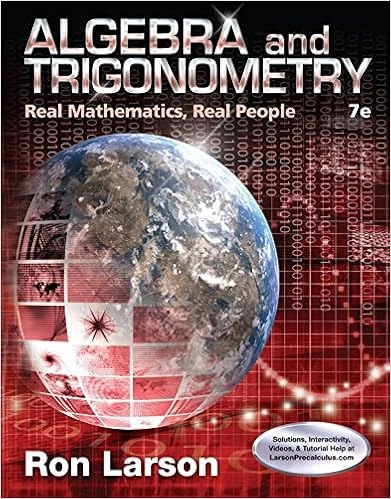# Linear Algebra with Applications (3rd Edition)

• Homework Help
• davidvictor
• 9
• 100% (2) 2 out of 2 people found this document helpful

This preview shows page 7 - 9 out of 9 pages.

##### We have textbook solutions for you!
The document you are viewing contains questions related to this textbook.The document you are viewing contains questions related to this textbook.
Chapter 4 / Exercise 63
Algebra and Trigonometry: Real Mathematics, Real People
LarsonExpert Verified
6b. [3 marks] For what real values of c (if any) is A = - 1 c 2 c - 4 - 3 2 - 3 4 a symmetric positive definite matrix? 6c. [4 marks] Let A = 3 4 4 3 . Is the quadratic form q ( x, y ) associated to A posi- tive definite? Find its principal axes. Sol. [6a.] Not possible. The condition means that B = 1 1 1 1 2 2 1 2 2 is similar to A = 1 1 1 1 1 1 1 1 1 . Similar matrices have equal traces and rank. But trace( A ) = 3 6 = trace( B ) = 5 and rk( A ) = 1 6 = rk( B ) = 2. [6b.] Not possible. For symmetric positive-definite matrices all upper-left determi- nants are greater than zero. Note that the 1 by 1 upper-left determinant is - 1. [6c.] det( A ) < 0, hence A (or equivalently its associated quadratic form q ( x, y ) = 3 x 2 + 8 xy + 3 y 2 ) is not positive definite. The eigenvalues of A are: λ 1 = - 1 and λ 2 = 7. The principal axes are the eigenspaces of A , namely E 1 = span { - 1 1 } and E 2 = span { 1 1 } .
##### We have textbook solutions for you!
The document you are viewing contains questions related to this textbook.The document you are viewing contains questions related to this textbook.
Chapter 4 / Exercise 63
Algebra and Trigonometry: Real Mathematics, Real People
LarsonExpert Verified
7. [15 marks] 7a. [6 marks] Let A 1 = 0 - 1 0 - 1 0 0 0 0 2 . Is A 1 diagonalizable? Why? Is A 1 in- vertible? Why? Determine the spectral decomposition of A 1 into projection matrices. 7b. [3 marks] Let A 2 = - 3 3 1 - 1 . Is A 2 invertible? Why? Is A 2 diagonalizable? Why? Determine (if exists) a matrix S and a diagonal matrix D such that S - 1 A 2 S = D . 7c.[6 marks]Describe the linear transformationTA2:R2R2associated toA2.Does suchA2have a decomposition into projection matrices? If yes, give it. 1. It follows that the spectral decomposition of A 1 is A 1 = 2 P 1 + P 2 - P 3 = 2 0 0 0 0 0 0 0 0 1 + 1 - 1 0 - 1 1 0 0 0 0 - 1 1 0 1 1 0 0 0 0 . [7b.] det( A 2 ) = 0 hence A 2 is not invertible. The eigenvalues of A 2 are μ 1 = 0 and μ 2 = - 4. They are distinct, hence A 2 is diagonalizable. The columns of the matrix S are made by 2 eigenvectors of A i.e. S = 1 - 3 1 1 , whereas D = 0 0 0 - 4 is the eigenvalues matrix. [7c.] The linear transformation T A 2 : R 2 R 2 is determined by a projection P 2 onto the line spanned by - 3 1 A 2 1 1 = 0 , A 2 - 3 1 = - 4 - 3 1 .
•••